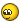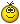We use cookies to ensure that we give you the best experience on our website. If you continue to use this site we will assume that you are happy with it.

# Converting hex to rgb for vertex colors. (Got it!)

Home Forums WebGL & WebXR Troubleshooting Converting hex to rgb for vertex colors. (Got it!)

Viewing 8 posts - 1 through 8 (of 8 total)
• Author
Posts
• #26461

I realize that there is a way to input hex into the material colors but there doesn’t seem to be a clear way to set vertex color that way. I found a code snippet and added it to the JS then plugged it in to the color picker target:

``````app.ExternalInterface.hexToRgb = function(hex) {
// Expand shorthand form (e.g. "03F") to full form (e.g. "0033FF")
var shorthandRegex = /^#?([a-f\d])([a-f\d])([a-f\d])\$/i;
hex = hex.replace(shorthandRegex, function(m, r, g, b) {
return r + r + g + g + b + b;
});

var result = /^#?([a-f\d]{2})([a-f\d]{2})([a-f\d]{2})\$/i.exec(hex);
return result ? {
r: parseInt(result, 16),
g: parseInt(result, 16),
b: parseInt(result, 16)
} : null;

}``````

The result is not formatted in a way I can use, I need just the digits (228,60,31) or better yet linear (0.894,0.2353,0.1216)

``````Object { r: 228, g: 60, b: 31 }
visual_logic.js:610:11``````

I then plug that into the math puzzles to /255. Gamma 2.2 is handled in the shader nodes.

If anyone can help I’d really appreciate input.

Edit: Or maybe if input type=”color” can output rgb.

###### Attachments:
You must be logged in to view attached files.
#26479

I found a shorter snippet that works perfectly:

``````app.ExternalInterface.hexToRgb = function(hex) {
return ['0x' + hex + hex | 0, '0x' + hex + hex | 0, '0x' + hex + hex | 0];
}``````

Edit: Need to check more.

#26484

This is the code that ended up working for me:

``````function hex2rgb(hex, opacity) {
var h=hex.replace('#', '');
h =  h.match(new RegExp('(.{'+h.length/3+'})', 'g'));

for(var i=0; i<h.length; i++)
h = parseInt(h.length==1? h+h:h, 16);

if (typeof opacity != 'undefined')  h.push(opacity);

return 'rgba('+h.join(',')+')';
}``````
#26487

https://tinyurl.com/ybmfd5yq

#26558

are you supposed to be able to also feed a Hex value into the sphere’s clicked prompt window that asks for RBG?

#26560

No, just the color picker in the top left. The RGB on the spheres was before I built the html with color picker.

#26600

glad you worked it out!3D Verger, the CEO
Hi @Xen, Would you mind to explain little bit more on this ?